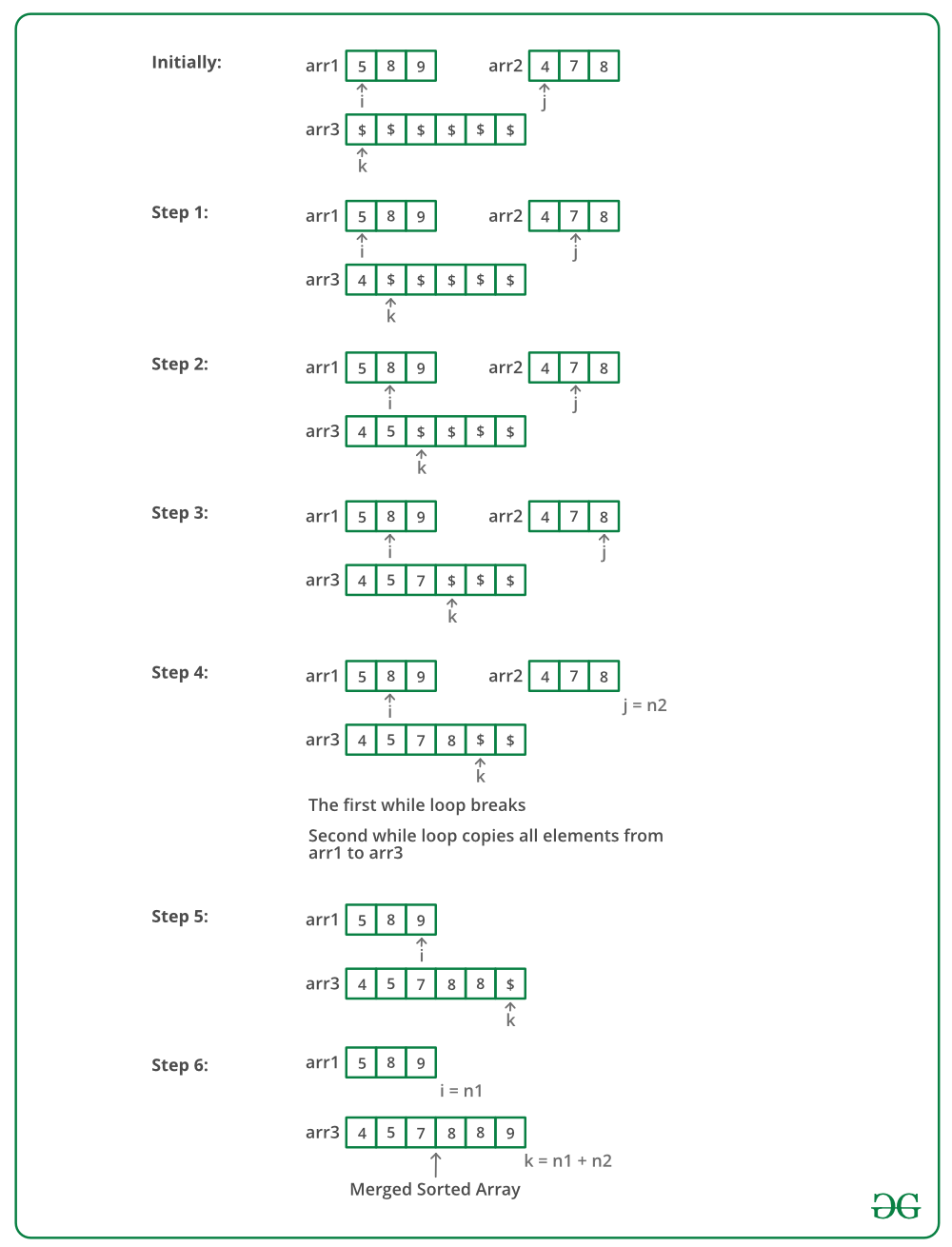Open in App
Not now

# C++ Program to Merge Two Sorted Arrays

• Last Updated : 17 Jan, 2023

Given two sorted arrays, the task is to merge them in a sorted manner.
Examples:

Input: arr1[] = { 1, 3, 4, 5}, arr2[] = {2, 4, 6, 8}
Output: arr3[] = {1, 2, 3, 4, 4, 5, 6, 8}

Input: arr1[] = { 5, 8, 9}, arr2[] = {4, 7, 8}
Output: arr3[] = {4, 5, 7, 8, 8, 9}

Naive Approach:

It is the brute force method to do the same. Take all the elements of arr1 and arr2 in arr3. Then simply sort the arr3.

The implementation of above approach is:

## C++

 `// C++ program to merge two sorted arrays/ ` `#include ` `using` `namespace` `std; ` ` `  `void` `mergeArrays(``int` `arr1[], ``int` `arr2[], ``int` `n1, ` `                            ``int` `n2, ``int` `arr3[]) ` `{ ` `    ``int` `i = 0, j = 0, k = 0; ` `      ``// traverse the arr1 and insert its element in arr3 ` `      ``while``(i < n1){ ` `      ``arr3[k++] = arr1[i++]; ` `    ``} ` `       `  `      ``// now traverse arr2 and insert in arr3 ` `      ``while``(j < n2){ ` `      ``arr3[k++] = arr2[j++]; ` `    ``} ` `       `  `      ``// sort the whole array arr3 ` `      ``sort(arr3, arr3+n1+n2); ` `} ` ` `  `// Driver code ` `int` `main() ` `{ ` `    ``int` `arr1[] = {1, 3, 5, 7}; ` `    ``int` `n1 = ``sizeof``(arr1) / ``sizeof``(arr1); ` ` `  `    ``int` `arr2[] = {2, 4, 6, 8}; ` `    ``int` `n2 = ``sizeof``(arr2) / ``sizeof``(arr2); ` ` `  `    ``int` `arr3[n1+n2]; ` `    ``mergeArrays(arr1, arr2, n1, n2, arr3); ` ` `  `    ``cout << ``"Array after merging"` `<

Output

```Array after merging
1 2 3 4 5 6 7 8 ```

Time Complexity : O((m+n) log(m+n)) , the whole size of arr3 is m+n
Auxiliary Space: O(1), No extra space is used

Method 2 (O(n1 * n2) Time and O(n1+n2) Extra Space)

1. Create an array arr3[] of size n1 + n2.
2. Copy all n1 elements of arr1[] to arr3[]
3. Traverse arr2[] and one by one insert elements (like insertion sort) of arr3[] to arr1[]. This step take O(n1 * n2) time.

We have discussed implementation of above method in Merge two sorted arrays with O(1) extra space
Method 3 (O(n1 + n2) Time and O(n1 + n2) Extra Space)
The idea is to use Merge function of Merge sort

1. Create an array arr3[] of size n1 + n2.
2. Simultaneously traverse arr1[] and arr2[].
• Pick smaller of current elements in arr1[] and arr2[], copy this smaller element to next position in arr3[] and move ahead in arr3[] and the array whose element is picked.
3. If there are remaining elements in arr1[] or arr2[], copy them also in arr3[].

Below image is a dry run of the above approach:Below is the implementation of the above approach:

## C++

 `// C++ program to merge two sorted arrays/ ` `#include ` `using` `namespace` `std; ` ` `  `// Merge arr1[0..n1-1] and arr2[0..n2-1] into ` `// arr3[0..n1+n2-1] ` `void` `mergeArrays(``int` `arr1[], ``int` `arr2[], ``int` `n1, ` `                             ``int` `n2, ``int` `arr3[]) ` `{ ` `    ``int` `i = 0, j = 0, k = 0; ` ` `  `    ``// Traverse both array ` `    ``while` `(i

Output

```Array after merging
1 2 3 4 5 6 7 8 ```

Output:

```Array after merging
1 2 3 4 5 6 7 8```

Time Complexity : O(n1 + n2)
Auxiliary Space : O(n1 + n2)

Method 4: Using Maps (O(nlog(n) + mlog(m)) Time and O(N) Extra Space)

1. Insert elements of both arrays in a map as keys.
2. Print the keys of the map.

Below is the implementation of above approach.

## CPP

 `// C++ program to merge two sorted arrays  ` `//using maps  ` `#include ` `using` `namespace` `std; ` ` `  `// Function to merge arrays ` `void` `mergeArrays(``int` `a[], ``int` `b[], ``int` `n, ``int` `m)  ` `{ ` `    ``// Declaring a map. ` `    ``// using map as a inbuilt tool ` `    ``// to store elements in sorted order. ` `    ``map<``int``, ``int``> mp; ` `     `  `    ``// Inserting values to a map. ` `    ``for``(``int` `i = 0; i < n; i++)mp[a[i]]++; ` `     `  `     `  `    ``for``(``int` `i = 0;i < m;i++)mp[b[i]]++; ` ` `  `     `  `    ``// Printing keys of the map. ` `    ``for``(``auto` `j: mp) ` `    ``{ ` `         ``for``(``int` `i=0; i

Output

`1 2 3 4 5 6 7 8 `

Time Complexity: O( nlog(n) + mlog(m) )
Auxiliary Space: O(N)